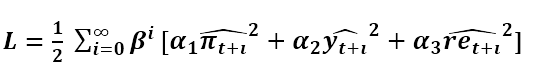# Ramsey policy in linear model

Hi everyone

1- Is it possible to apply Ramsey policy in a “linear DSGE model”? are the results of the approximated value of the planner objective function make sense in a linear DSGE model or we should use Ramsey policy just in a nonlinear model?

2- Is there a difference between the interpretation of results in linear and nonlinear DSGE models?

3- can I use Ramsey policy without an instrument? is it correct? what is the difference between the interpretation of the value of the planner objective function with and without the instrument?

4- can we calculate the value of the planner objective function without Ramsey policy command in the model?

5- is the function like "" is correct in a linear DSGE model as a planner objective function that follows a linear quadratic composition of main variables in the form of difference from its steady state?

6- when we want to use utility function as a planner objective function in a linear model, substituting the above function in question 5, which form of utility function should be used? linear form or nonlinear?

1. Yes that is possible. The question is whether a linear model is sufficient to capture the welfare effects. That may depend on whether your steady state is distorted or not.
2. See the response to 1. You may get a wrong welfare ranking if the true model is nonlinear and you approximate it only linearly.
3. The difference is only computationally. If you specify an instrument together with a steady state file, it makes finding the steady state of the system a lot easier.
4. No, that is not possible, because it introduces a singularity.
5. It’s not clear what you mean here. If that is the planner objective it is the planner objective. Why would it be wrong?
6. The utility function to be used is of course the original nonlinear one. Even your approximated objective was quadratic.

thanks a lot, Mr. Pfeifer for your responses
i’m so sorry. i think that i asked some of my question badly and inaccurate

1- what do you mean about “That may depend on whether your steady state is distorted or not” in case 1? distortion from what?

3- are the steady-state values different in the Ramsey monetary policy and without it(just Taylor rule) in the nonlinear model? does using Ramsey policy, change the steady state values of variables? What about the linear model?

4- I want to compare the welfare effect of using Ramsey monetary policy vs Taylor monetary policy in a “linear model”. dynare calculates the value of objective function according to the objective function of planner that is 0.006. how can I calculate welfare in the Taylor policy to compare with 0.006? does dynare calculate that value or i should calculate manually?
can i use Welf = Utility + beta*Welf(+1) in the model (offcours in the linear form and linear form of utility) for calculation the value of welfare in Taylor rule? if yes, should I compare the mean of Welf with 0.006 or the primary of Welf in t=0?

5- in my linear model, I use Ramsey monetary policy with instrument of i (nominal interest rate) and the value of planner objective function is 0.07. when I remove the instrument, the value of planner objective function is 0.08. why do these values differ? what happens when we use an instrument?

6- i want to use nonlinear utility function as planner objective function in Ramsey policy in a “linear model” instead of linear composition quadratic form of some variables. is it possible? if not, should i use linear form of utility func. as planner objective function?

Why do you even care about linear or nonlinear? If you set order=1, Dynare will take a linear approximation even if you enter a nonlinear equation.

1. It can for example be monopolistic competition. Please have a look at Woodford’s 2002 paper “Inflation Stabilization and Welfare”
2. There is no general answer here. The Taylor rule with its inflation target selects a particular steady state for inflation. Ramsey may find that a different inflation rate may be optimal.
3. You should be able to implement exactly the same objective function in your non-Ramsey model.
4. Your objective is a loss function, so the lower the better. As I said, using an instrument makes the problem computationally simpler. It seems the non-instrument version got stuck at a different steady state with worse welfare properties.
5. Of course that is possible. See https://git.dynare.org/Dynare/dynare/-/blob/master/examples/Ramsey_Example.mod

thanks, Mr. Pfeifer
according to your answer 3, how can I implement an objective function in my non-Ramsey model? do you mean, I should add the same objective function of Ramsey model as one of my equations? If so, where is the amount of welfare stored for comparing to value of objective fun. in Ramsey model?

according to your response 5, my model is linear but the model in your offered example is nonlinear and it’s accurately my question. can I use a nonlinear form of utility fun. as an objective fun. in a “”“linear model”""? otherwise, can i use a linear form of utility fun as objective function?

in this post,

are your solution for ξ is appropriate and correct in a linear model? can i calculate ξ as compensated variation in a “”“linear model”""" or this solution is especially for nonlinear model? how can i compute ξ in a linear model whereas steady state value for c and h is zero?

This depends very much on whether your policy will affect the steady state of the economy or not. If the steady state is affected, then expressing everything in deviations from a steady state that differs will be misleading.

I keep repeating myself. You cannot work with a linear utility function. But you may be able to express your nonlinear utility function in variables that measure deviations from steady state.

Related: